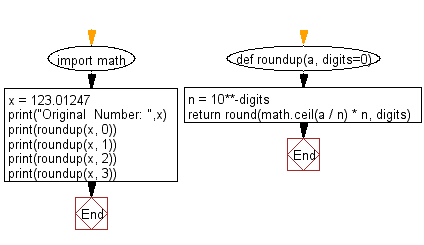﻿ Python Math: Round up a number to specified digits - w3resourcePython Math: Round up a number to specified digits

Python Math: Exercise-69 with Solution

Write a Python function to round up a number to specified digits.

Sample Solution:-

Python Code:

import math

def roundup(a, digits=0):
n = 10**-digits
return round(math.ceil(a / n) * n, digits)

x = 123.01247
print("Original  Number: ",x)
print(roundup(x, 0))
print(roundup(x, 1))
print(roundup(x, 2))
print(roundup(x, 3))

Sample Output:

Original  Number:  123.01247
124
123.1
123.02
123.013

Flowchart:Visualize Python code execution:

The following tool visualize what the computer is doing step-by-step as it executes the said program:

Python Code Editor:

Have another way to solve this solution? Contribute your code (and comments) through Disqus.

What is the difficulty level of this exercise?

Test your Programming skills with w3resource's quiz.

﻿

Python: Tips of the Day

Memory Management:

getrefcount will show how many times an object is used in the memory. It's a fantastic tool that can be used for memory management in any program and it's very convenient too.

Getrefcount will calculate the object usage at a low level ByteCode so it can tend to be higher than expected. For instance when you print a value that value is actually processed multiple times in the background inside the print function itself and getrefcount also counts the instance when the value is called with getrefcount method itself. So, it's safe to say that the count will actually always be at least 1 time higher than expected.

Here is a code to show how many times alphanumeric characters are referenced in a random code:

import sys
x_val = 20
x_val += 1

y_val = "Heritage"

x = sys.getrefcount(x_val)
y = sys.getrefcount(y_val)

print(x, y, sep="\n")

Output:

10
4